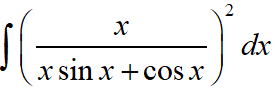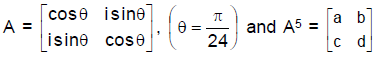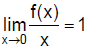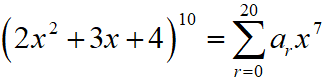Saturday, December 4, 2021
Home > IIT JEE Main and Advance > JEE Main 2020 Question Paper Answer Keys Solutions 4 September Morning Shift

# JEE Main 2020 Question Paper Answer Keys Solutions 4 September Morning Shift

Hi Students, welcome to Amans Maths Blogs (AMB). On this post, you will get JEE Main 2020 Question Paper Answer Keys Solutions 4 September Morning Shift. As you know that Joint Entrance Examination means JEE Main and JEE Advanced is one of the most important entrance exam in engineering entrance examination. JEE Main 2020 is Computer Based Test (CBT) and it was conducted by the National Testing Agency (NTA). JEE Main 2020 exam duration is three hours and the exam consists of 300 marks (Maximum Marks). JEE Main 2020 Questions Paper has 2 sections in all three Subject Papers (Physics, Chemistry, Mathematics) and each subject has 25 questions.

## JEE Main 2020 Physics Questions Paper with Answer Keys & Solutions

In JEE Main 2020 Question Paper Answer Keys Solutions 4 September Morning Shift, Physics question paper’s answer keys and solutions by Resonance.

JEE Main 2020 Physics Question Paper with Answer Keys and Solutions (By Resonance)

## JEE Main 2020 Chemistry Questions Paper with Answer Keys & Solutions

In JEE Main 2020 Question Paper Answer Keys Solutions 4 September Morning Shift, Chemistry question paper’s answer keys and solutions by Resonance.

JEE Main 2020 Chemistry Question Paper with Answer Keys and Solutions (By Resonance)

## JEE Main 2020 Mathematics Questions Paper with Answer Keys & Solutions

In JEE Main 2020 Question Paper Answer Keys Solutions 4 September Morning Shift, Mathematics question paper’s answer keys and solutions by Amans Maths Blogs (AMB).

Instructions for SECTION 1 : (Maximum Marks : 80):

This section contains 20 multiple choice questions. Each question has 4 choices (1), (2), (3) and (4) for its answer, out of which Only One is correct.

JEE Main 2020 Maths Questions Answer Keys Solutions 4th Sep Shift 1 : Ques No 1

Let y = y(x) be the solution of the differential equation, xy’ – y = x2(xcosx + sinx), x > 0. If y(π) = π, then y”(π) + y(π) is equal to

Options:

A. 1 + π/2

B. 1 + π/2 + π2/4

C. 2 + π/2 + π2/4

D. 2 + π/2

Solution:

JEE Main 2020 Mathematics Questions with Answer Keys and Solutions : Ques No 2

A triangle ABC lying in the first quadrant has two vertices as A(1, 2) and B(3, 1). If angle BAC = 90°, and ar(triangle ABC) = 5√5 sq. units, then the abscissa of the vertex C is :

Options:

A. 1 + √5

B. 2 +√5

C. 1 + 2√5

D. 2√5 – 1

Solution:

JEE Main 2020 Maths Questions Answer Keys Solutions 4th Sep Shift 1 : Ques No 3

Let f(x) = |x – 2| and g(x) = f(f(x)), x ∈ [0, 4]. Thenis equal to

Options:

A. 0

B. 1/2

C. 3/2

D. 1

Solution:

JEE Main 2020 Mathematics Questions with Answer Keys and Solutions : Ques No 4

The integralis equal to (where C is a constant of integration) :

Options:

A. tanx + xsecx / (xsinx + cosx) + C

B. tanx – xsecx / (xsinx + cosx) + C

C. secx + xtanx / (xsinx + cosx) + C

D. secx – xtanx / (xsinx + cosx) + C

Solution:

JEE Main 2020 Maths Questions Answer Keys Solutions 4th Sep Shift 1 : Ques No 5

If 1 + (1 – 22.1) + (1 – 42.3) + (1 – 62.5) + …… + (1 – 202.19) = α – 220β then an ordered pair (α, β) is equal to

Options:

A. (11, 97)

B. (10, 103)

C. (11, 103)

D. (10, 97)

Solution:

JEE Main 2020 Mathematics Questions with Answer Keys and Solutions : Ques No 6

A survey shows that 63% of the people in a city read newspaper A whereas 76% read news paper B. If x% of the people read both the newspapers, then a possible value of x can be :

Options:

A. 65

B. 55

C. 37

D. 29

Solution:

JEE Main 2020 Maths Questions Answer Keys Solutions 4th Sep Shift 1 : Ques No 7

Two vertical poles AB = 15 m and CD = 10 m are standing apart on a horizontal ground with points A and C on the ground. If P is the point of intersection of BC and AD, then the height of P (in m) above the line AC is :

Options:

A. 10/3

B. 6

C. 5

D. 20/3

Solution:

JEE Main 2020 Mathematics Questions with Answer Keys and Solutions : Ques No 8

Let P(3, 3) be a point on the hyperbola x2 / a2 – y2 / b2 = 1. If the normal to it at P intersects the x-axis at (9,0) and e is its eccentricity, then the ordered pair (a2, e2) is equal to :

Options:

A. (9/2, 3)

B. (3/2, 2)

C. (9, 3)

D. (9/2, 2)

Solution:

JEE Main 2020 Maths Questions Answer Keys Solutions 4th Sep Shift 1 : Ques No 9

Let [t] denote the greatest integer ≤ t. Then the equation in x, [x]2 + 2[x + 2] – 7 = 0 has :

Options:

A. no integral solution

B. infinitely many solutions

C. exactly two solutions

D. exactly four integral solutions

Solution:

JEE Main 2020 Mathematics Questions with Answer Keys and Solutions : Ques No 10

If (a + √2 bcosx)(a – √2 bcosy) = a2 – b2, where a > b > 0, then dx/dy at (π/4, π/4) is

Options:

A. (2a + b) / (2a – b)

B. (a + b) / (a – b)

C. (a – b) / (a + b)

D. (a – 2b) / (a + 2b)

Solution:

JEE Main 2020 Maths Questions Answer Keys Solutions 4th Sep Shift 1 : Ques No 11

Let x0 be the point of local maxima of f(x) = a.(b x c), where a = xi – 2j + 3k, b = -2i + xjk and c = 7i – 2j + xk. Then, the value of a.b + b.c + c.a at x = x0 is

Options:

A. -22

B. 14

C. -4

D. -30

Solution:

JEE Main 2020 Mathematics Questions with Answer Keys and Solutions : Ques No 12

Let, Then f(3) – f(1) is equal to :

Options:

A. -π/6 + 1/2 + √3/4

B. -π/12 + 1/2 + √3/4

C. π/12 + 1/2 – √3/4

D. π/6 + 1/2 – √3/4

Solution:

JEE Main 2020 Maths Questions Answer Keys Solutions 4th Sep Shift 1 : Ques No 13

The mean and variance of 8 observations are 10 and 13.5, respectively. If 6 of these observations are 5, 7, 10, 12 , 14, 15, then the absolute difference of the remaining two observations is

Options:

A. 9

B. 5

C. 3

D. 7

Solution:

JEE Main 2020 Mathematics Questions with Answer Keys and Solutions : Ques No 14

Let x2/a2 + y2/b2 = 1 (a > b) be a given ellipse, length of whose latus rectum is 10. If its eccentricity is the maximum value of the function φ(t) = 5/12 + t – t2, then a2 + b2 is equal to

Options:

A. 145

B. 126

C. 116

D. 135

Solution:

JEE Main 2020 Maths Questions Answer Keys Solutions 4th Sep Shift 1 : Ques No 15

Let f be a twice differentiable function on (1, 6). If f(2) = 8, f'(2) = 5, f'(x) ≥ 1 and f”(x) ≥ 4, for all x ∈ (1, 6), then :

Options:

A. f(5) + f'(5) ≥ 28

B. f'(5) + f”(5) ≤ 20

C. f(5) ≤ 10

D. f(5) + f'(5) ≤ 26

Solution:

JEE Main 2020 Mathematics Questions with Answer Keys and Solutions : Ques No 16

Let u = (2z + i) / (z – ki), z = x + iy and k > 0. If the curve represented by Re(u) + Im(u) = 1 intersects the y-axis at points P and Q where PQ = 5 then the value of k is

Options:

A. 3/2

B. 1/2

C. 4

D. 2

Solution:

JEE Main 2020 Maths Questions Answer Keys Solutions 4th Sep Shift 1 : Ques No 17

If, where i = √(-1) then which one of the following is
not true ?

Options:

A. 0 ≤ a2 + b2 ≤ 1

B. a2 – d2 = 0

C. a2 – b2 = 1/2

D. a2 – c2 = 1

Solution:

JEE Main 2020 Mathematics Questions with Answer Keys and Solutions : Ques No 18

Let α and β be the roots of x2 – 3x + p = 0 and ? and δ be the roots of x2 – 6x + q = 0. If α, β,  ?, δ from a geometric progression. Then ratio (2q + p) : (2q – p) is

Options:

A. 3 : 1

B. 5 : 3

C. 9 : 7

D. 33 : 31

Solution:

JEE Main 2020 Maths Questions Answer Keys Solutions 4th Sep Shift 1 : Ques No 19

The value ofis equal to

Options:

A. 51C30C7

B. 51C+ 30C7

C. 50C30C7

D. 50C6 30C6

Solution:

JEE Main 2020 Mathematics Questions with Answer Keys and Solutions : Ques No 20

Given the following two statements :

(S1) : (q V p) → (p ↔ ~q) is a tautology.

(S2) : ~q ∧ (~p ↔ q) is a fallacy. Then

Options:

A. Only S2 is correct

B. Both S1 and S2 are NOT correct

C. Both S1 and S2 are correct

D. Only S1 is correct

Solution:

Instructions for SECTION 2 : (Maximum Marks : 20):

This section contains FIVE (05) questions. The answer to each question is NUMERICAL VALUE with two digit integer and decimal upto one digit.

If the numerical value has more than two decimal places truncate/round-off the value upto TWO decimal places.

Full Marks : +4 If ONLY the correct option is chosen

Zero Marks : 0 In all other cases

JEE Main 2020 Maths Questions Answer Keys Solutions 4th Sep Shift 1 : Ques No 21

If the equation of a plane P, passing through the intersection of the planes, x + 4y – z + 7 = 0 and
3x + y + 5z = 8 is ax + by + 6z = 15 for some a, b ∈ R, then the distance of the point (3, 2, –1) from the plane P is ……

Solution:

JEE Main 2020 Mathematics Questions with Answer Keys and Solutions : Ques No 22

Suppose a differentiable function f(x) satisfies the identity f(x + y) = f(x) + f(y) + xy2 + x2y, for all real x and y. If, then f'(3) is equal to :

Solution:

JEE Main 2020 Maths Questions Answer Keys Solutions 4th Sep Shift 1 : Ques No 23

The probability of a man hitting a target is 1/10. The least number of shots required, so that the
probability of his hitting the target at least once is greater than 1/4, is …

Solution:

JEE Main 2020 Mathematics Questions with Answer Keys and Solutions : Ques No 24

Let, then a7/a13 is equal to

Solution:

JEE Main 2020 Maths Questions Answer Keys Solutions 4th Sep Shift 1 : Ques No 25

If the system of equations
x – 2y + 3z = 9
2x + y + z = b
x – 7y + az = 24, has infinitely many solutions, then a – b is equal to :